Create a new printableMath Worksheets

Sample - Click above to make a new math worksheet (PDF).
 Name _____________________________Date ___________________
Write the sum or difference.

1.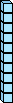-7 tens  -  3 tens

=  ___ tens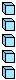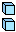-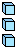7 ones  -  3 ones

=  ___ ones

77  -  33  =  ______
2.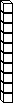-4 tens  -  1 ten

=  ___ tens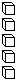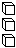-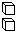8 ones  -  2 ones

=  ___ ones

48  -  12  =  ______
3.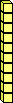+2 tens  +  1 ten

=  ___ tens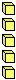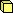+6 ones  +  1 one

=  ___ ones

26  +  11  =  ______
4.-7 tens  -  4 tens

=  ___ tens-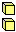6 ones  -  2 ones

=  ___ ones

76  -  42  =  ______
 5 * This is a pre-made sheet.Use the link at the top of the page for a printable page.
6.
 +tens  +  3 tens

=  ___ tens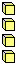+4 ones  +  1 one

=  ___ ones

4  +  31  =  ______